# SciPy-数值计算库

SciPy函数库在NumPy库的基础上增加了众多的数学、科学以及工程计算中常用的库函数。例如线性代数、常微分方程数值求解、信号处理、图像处理、稀疏矩阵等等。由于其涉及的领域众多、本书没有能力对其一一的进行介绍。作为入门介绍，让我们看看如何用SciPy进行插值处理、信号滤波以及用C语言加速计算。

## 最小二乘拟合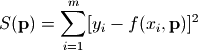scipy中的子函数库optimize已经提供了实现最小二乘拟合算法的函数leastsq。下面是用leastsq进行数据拟合的一个例子：

  1 2 3 4 5 6 7 8 9 10 11 12 13 14 15 16 17 18 19 20 21 22 23 24 25 26 27 28 29 30 31 32 33 34 35 36 37 38 39 # -*- coding: utf-8 -*- import numpy as np from scipy.optimize import leastsq import pylab as pl def func(x, p): """ 数据拟合所用的函数: A*sin(2*pi*k*x + theta) """ A, k, theta = p return A*np.sin(2*np.pi*k*x+theta) def residuals(p, y, x): """ 实验数据x, y和拟合函数之间的差，p为拟合需要找到的系数 """ return y - func(x, p) x = np.linspace(0, -2*np.pi, 100) A, k, theta = 10, 0.34, np.pi/6 # 真实数据的函数参数 y0 = func(x, [A, k, theta]) # 真实数据 y1 = y0 + 2 * np.random.randn(len(x)) # 加入噪声之后的实验数据 p0 = [7, 0.2, 0] # 第一次猜测的函数拟合参数 # 调用leastsq进行数据拟合 # residuals为计算误差的函数 # p0为拟合参数的初始值 # args为需要拟合的实验数据 plsq = leastsq(residuals, p0, args=(y1, x)) print u"真实参数:", [A, k, theta] print u"拟合参数", plsq # 实验数据拟合后的参数 pl.plot(x, y0, label=u"真实数据") pl.plot(x, y1, label=u"带噪声的实验数据") pl.plot(x, func(x, plsq), label=u"拟合数据") pl.legend() pl.show() 

>>> 真实参数: [10, 0.34000000000000002, 0.52359877559829882]
>>> 拟合参数 [-9.84152775  0.33829767 -2.68899335]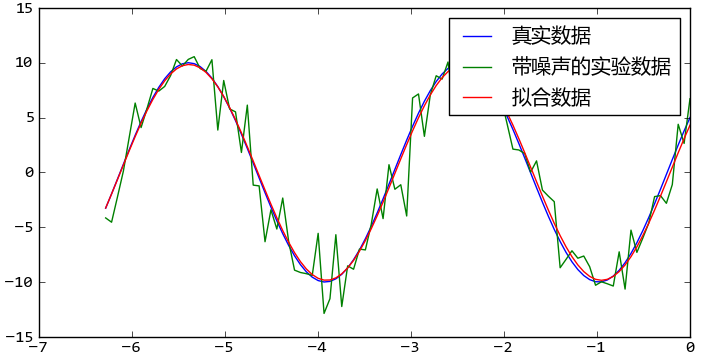## 函数最小值

optimize库提供了几个求函数最小值的算法：fmin, fmin_powell, fmin_cg, fmin_bfgs。下面的程序通过求解卷积的逆运算演示fmin的功能。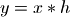1 2 3 4 5 6 7 8 9 10 11 12 13 14 15 16 17 18 19 20 21 22 23 24 25 26 27 28 29 30 31 32 33 34 35 36 37 38 39 40 41 42 43 44 45 46 47 48 # -*- coding: utf-8 -*- # 本程序用各种fmin函数求卷积的逆运算 import scipy.optimize as opt import numpy as np def test_fmin_convolve(fminfunc, x, h, y, yn, x0): """ x (*) h = y, (*)表示卷积 yn为在y的基础上添加一些干扰噪声的结果 x0为求解x的初始值 """ def convolve_func(h): """ 计算 yn - x (*) h 的power fmin将通过计算使得此power最小 """ return np.sum((yn - np.convolve(x, h))**2) # 调用fmin函数，以x0为初始值 h0 = fminfunc(convolve_func, x0) print fminfunc.__name__ print "---------------------" # 输出 x (*) h0 和 y 之间的相对误差 print "error of y:", np.sum((np.convolve(x, h0)-y)**2)/np.sum(y**2) # 输出 h0 和 h 之间的相对误差 print "error of h:", np.sum((h0-h)**2)/np.sum(h**2) print def test_n(m, n, nscale): """ 随机产生x, h, y, yn, x0等数列，调用各种fmin函数求解b m为x的长度, n为h的长度, nscale为干扰的强度 """ x = np.random.rand(m) h = np.random.rand(n) y = np.convolve(x, h) yn = y + np.random.rand(len(y)) * nscale x0 = np.random.rand(n) test_fmin_convolve(opt.fmin, x, h, y, yn, x0) test_fmin_convolve(opt.fmin_powell, x, h, y, yn, x0) test_fmin_convolve(opt.fmin_cg, x, h, y, yn, x0) test_fmin_convolve(opt.fmin_bfgs, x, h, y, yn, x0) if __name__ == "__main__": test_n(200, 20, 0.1) 

fmin
ーーーーーーーーーーー
error of y: 0.00568756699607
error of h: 0.354083287918

fmin_powell
ーーーーーーーーーーー
error of y: 0.000116114709857
error of h: 0.000258897894009

fmin_cg
ーーーーーーーーーーー
error of y: 0.000111220299615
error of h: 0.000211404733439

fmin_bfgs
ーーーーーーーーーーー
error of y: 0.000111220251551
error of h: 0.000211405138529


## 非线性方程组求解

optimize库中的fsolve函数可以用来对非线性方程组进行求解。它的基本调用形式如下：

fsolve(func, x0)


func(x)是计算方程组误差的函数，它的参数x是一个矢量，表示方程组的各个未知数的一组可能解，func返回将x代入方程组之后得到的误差；x0为未知数矢量的初始值。如果要对如下方程组进行求解的话：

• f1(u1,u2,u3) = 0
• f2(u1,u2,u3) = 0
• f3(u1,u2,u3) = 0

def func(x):
u1,u2,u3 = x
return [f1(u1,u2,u3), f2(u1,u2,u3), f3(u1,u2,u3)]


• 5*x1 + 3 = 0
• 4*x0*x0 - 2*sin(x1*x2) = 0
• x1*x2 - 1.5 = 0

  1 2 3 4 5 6 7 8 9 10 11 12 13 14 15 16 17 from scipy.optimize import fsolve from math import sin,cos def f(x): x0 = float(x) x1 = float(x) x2 = float(x) return [ 5*x1+3, 4*x0*x0 - 2*sin(x1*x2), x1*x2 - 1.5 ] result = fsolve(f, [1,1,1]) print result print f(result) 

[-0.70622057 -0.6        -2.5       ]
[0.0, -9.1260332624187868e-14, 5.3290705182007514e-15]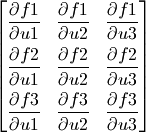1 2 3 4 5 6 7 8 9 10 11 12 13 14 15 16 17 18 19 20 21 22 23 24 25 26 # -*- coding: utf-8 -*- from scipy.optimize import fsolve from math import sin,cos def f(x): x0 = float(x) x1 = float(x) x2 = float(x) return [ 5*x1+3, 4*x0*x0 - 2*sin(x1*x2), x1*x2 - 1.5 ] def j(x): x0 = float(x) x1 = float(x) x2 = float(x) return [ [0, 5, 0], [8*x0, -2*x2*cos(x1*x2), -2*x1*cos(x1*x2)], [0, x2, x1] ] result = fsolve(f, [1,1,1], fprime=j) print result print f(result) 

## B-Spline样条曲线

interpolate库提供了许多对数据进行插值运算的函数。下面是使用直线和B-Spline对正弦波上的点进行插值的例子。

  1 2 3 4 5 6 7 8 9 10 11 12 13 14 15 16 17 18 # -*- coding: utf-8 -*- import numpy as np import pylab as pl from scipy import interpolate x = np.linspace(0, 2*np.pi+np.pi/4, 10) y = np.sin(x) x_new = np.linspace(0, 2*np.pi+np.pi/4, 100) f_linear = interpolate.interp1d(x, y) tck = interpolate.splrep(x, y) y_bspline = interpolate.splev(x_new, tck) pl.plot(x, y, "o", label=u"原始数据") pl.plot(x_new, f_linear(x_new), label=u"线性插值") pl.plot(x_new, y_bspline, label=u"B-spline插值") pl.legend() pl.show()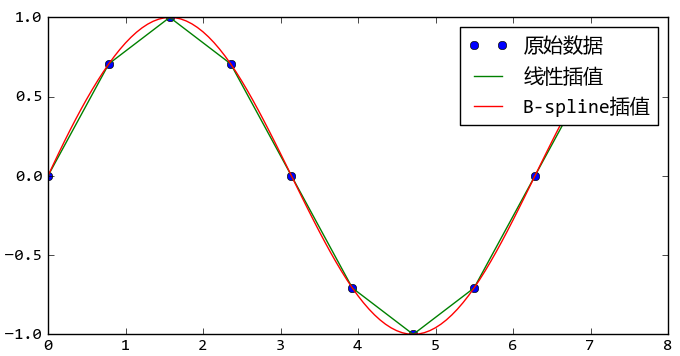## 数值积分

def half_circle(x):
return (1-x**2)**0.5


>>> N = 10000
>>> x = np.linspace(-1, 1, N)
>>> dx = 2.0/N
>>> y = half_circle(x)
>>> dx * np.sum(y[:-1] + y[1:]) # 面积的两倍
3.1412751679988937


>>> import numpy as np
>>> np.trapz(y, x) * 2 # 面积的两倍
3.1415893269316042


>>> from scipy import integrate
>>> pi_half, err = integrate.quad(half_circle, -1, 1)
>>> pi_half*2
3.1415926535897984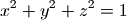def half_sphere(x, y):
return (1-x**2-y**2)**0.5


>>> integrate.dblquad(half_sphere, -1, 1,
lambda x:-half_circle(x),
lambda x:half_circle(x))
>>> (2.0943951023931988, 2.3252456653390915e-14)
>>> np.pi*4/3/2 # 通过球体体积公式计算的半球体积
2.0943951023931953


dblquad(func2d, a, b, gfun, hfun)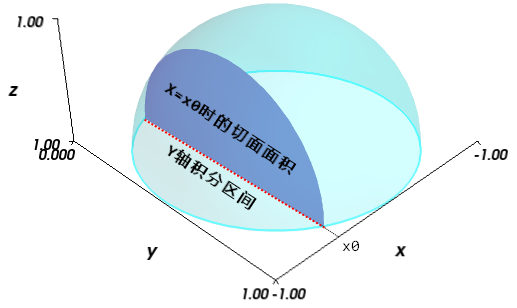X轴的积分区间为-1.0到1.0，对于X=x0时，通过对Y轴的积分计算出切面的面积，因此Y轴的积分区间如图中红色点线所示。

## 解常微分方程组

scipy.integrate库提供了数值积分和常微分方程组求解算法odeint。下面让我们来看看如何用odeint计算洛仑兹吸引子的轨迹。洛仑兹吸引子由下面的三个微分方程定义：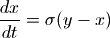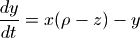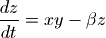1 2 3 4 5 6 7 8 9 10 11 12 13 14 15 16 17 18 19 20 21 22 23 24 25 # -*- coding: utf-8 -*- from scipy.integrate import odeint import numpy as np def lorenz(w, t, p, r, b): # 给出位置矢量w，和三个参数p, r, b计算出 # dx/dt, dy/dt, dz/dt的值 x, y, z = w # 直接与lorenz的计算公式对应 return np.array([p*(y-x), x*(r-z)-y, x*y-b*z]) t = np.arange(0, 30, 0.01) # 创建时间点 # 调用ode对lorenz进行求解, 用两个不同的初始值 track1 = odeint(lorenz, (0.0, 1.00, 0.0), t, args=(10.0, 28.0, 3.0)) track2 = odeint(lorenz, (0.0, 1.01, 0.0), t, args=(10.0, 28.0, 3.0)) # 绘图 from mpl_toolkits.mplot3d import Axes3D import matplotlib.pyplot as plt fig = plt.figure() ax = Axes3D(fig) ax.plot(track1[:,0], track1[:,1], track1[:,2]) ax.plot(track2[:,0], track2[:,1], track2[:,2]) plt.show()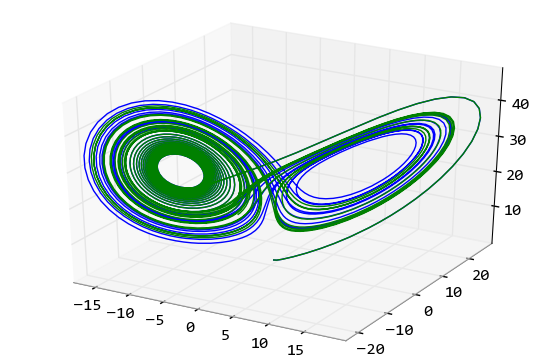1. lorenz， 它是计算某个位移上的各个方向的速度(位移的微分)
2. (0.0, 1.0, 0.0)，位移初始值。计算常微分方程所需的各个变量的初始值
3. t， 表示时间的数组，odeint对于此数组中的每个时间点进行求解，得出所有时间点的位置
4. args， 这些参数直接传递给lorenz函数，因此它们都是常量

## 滤波器设计

scipy.signal库提供了许多信号处理方面的函数。在这一节，让我们来看看如何利用signal库设计滤波器，查看滤波器的频率响应，以及如何使用滤波器对信号进行滤波。

>>> import scipy.signal as signal


>>> b, a = signal.iirdesign([0.2, 0.5], [0.1, 0.6], 2, 40)


iirdesgin返回的两个数组b和a， 它们分别是IIR滤波器的分子和分母部分的系数。其中a恒等于1。

>>> w, h = signal.freqz(b, a)


freqz返回两个数组w和h，其中w是圆频率数组，通过w/pi*f0可以计算出其对应的实际频率。h是w中的对应频率点的响应，它是一个复数数组，其幅值为滤波器的增益，相角为滤波器的相位特性。

>>> power = 20*np.log10(np.clip(np.abs(h), 1e-8, 1e100))


>>> pl.plot(w/np.pi*4000, power)


>>> t = np.arange(0, 2, 1/8000.0)


>>> sweep = signal.chirp(t, f0=0, t1 = 2, f1=4000.0)


>>> out = signal.lfilter(b, a, sweep)


lfilter内部通过如下算式计算IIR滤波器的输出：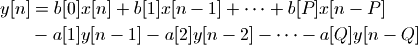>>> out = 20*np.log10(np.abs(out))


>>> index = np.where(np.logical_and(out[1:-1] > out[:-2], out[1:-1] > out[2:])) + 1


>>> pl.plot(t[index]/2.0*4000, out[index] )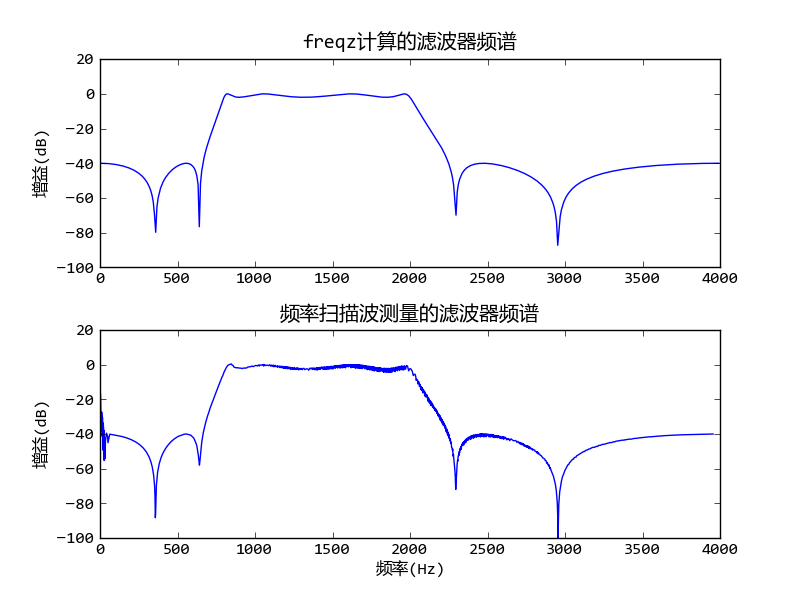## 用Weave嵌入C语言

Python作为动态语言其功能虽然强大，但是在数值计算方面有一个最大的缺点：速度不够快。在Python级别的循环和计算的速度只有C语言程序的百分之一。因此才有了NumPy, SciPy这样的函数库，将高度优化的C、Fortran的函数库进行包装，以供Python程序调用。如果这些高度优化的函数库无法实现我们的算法，必须从头开始写循环、计算的话，那么用Python来做显然是不合适的。因此SciPy提供了快速调用C++语言程序的方法-- Weave。下面是对NumPy的数组求和的例子：

  1 2 3 4 5 6 7 8 9 10 11 12 13 14 15 16 17 18 19 20 21 22 23 24 25 26 27 28 29 30 31 32 33 34 35 36 37 38 39 40 41 42 # -*- coding: utf-8 -*- import scipy.weave as weave import numpy as np import time def my_sum(a): n=int(len(a)) code=""" int i; double counter; counter =0; for(i=0;i

my_sum: 0.0294527349146
np.sum: 0.0527649547638
sum: 9.11022322669`

weave.inline函数的第一个参数为需要执行的C++语言代码，第二个参数是一个列表，它告诉weave要把Python中的两个变量a和n传递给C++程序，注意我们用字符串表示变量名。converters.blitz是一个类型转换器，将numpy的数组类型转换为C++的blitz类。C++程序中的变量a不是一个数组，而是blitz类的实例，因此它使用a(i)获得其各个元素的值，而不是用a[i]。最后我们通过compiler参数告诉weave要采用gcc为C++编译器。如果你安装的是python(x,y)的话，gcc(mingw32)也一起安装好了，否则你可能需要手工安装gcc编译器或者微软的Visual C++。

Note